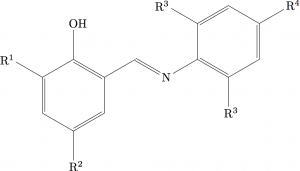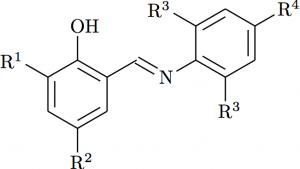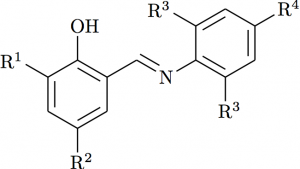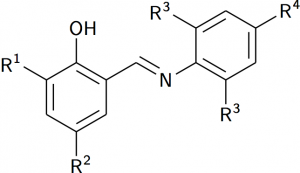In my previous post, I looked at the basics of using the ChemFig package to create chemical structures. I finished that post with a structure that is complete but which I think does not look great compared with the reference version I created in ChemDraw. (There’s a MyChemistry entry that looks at similar customisation: worth a look!)

## Atom placement

The first issue to tackle is the placement of atom labels. ChemFig ‘detects’ atoms, so that the labels are correctly centred relative to bonds. However, that does not work with numbered R-groups, as the numbers need to be ‘ignored’ for alignment purposes. This is a pretty common requirement, so ChemFig provides a way to ‘split’ labels, using |:

\chemfig{
*6(-(-R|^2)=-
(-=[::-60]N-*6(=(-R|^3)-=(-R|^4)-=(-R|^3)-))
=(-OH)-(-R|^1)=)
}


which gives the outputTo see the difference here, look at for example R2 here compared to the version in the previous post: it’s subtle, but it is there!

## Atom spacing and bond width

The standard settings for ChemFig share a ‘feature’ with those for ChemDraw: they don’t look very good! As I said in the previous post, I use the Royal Society of Chemistry’s template for my structures, as I think they look much better. The template uses 7 pt text, and so the lengths, etc. all match that size. For use in LaTeX, I want things to be more flexible so wanted to convert the values into em (i.e. relative dimensions based on font size).

There are three key dimensions used by both ChemDraw and ChemFig to set how bonds look: the bond length, the line width and the gap between lines when drawing double bonds. There is also a ‘margin’ used between atom labels and bonds, so the two don’t touch. After a bit of work doing the calculation (using to the LaTeX3 FPU), I found that

\setdoublesep{0.35700 em}  % 'Bond Spacing'
\setatomsep{1.78500 em}    % 'Fixed Length'
\setbondoffset{0.18265 em} % 'Margin Width'
\newcommand{\bondwidth}{0.06642 em} % 'Line Width'
\setbondstyle{line width = \bondwidth}


was the right set up. The comments are the ChemDraw names for settings, and I’ve set the line width as a command as it turns out I’ll want it again for some more advanced things to be covered in the next post.## The central double bond

Changing the bond spacing shows up another issue: the central double bond is not right. Rather than bond to the ‘middle’ of the double bond, we want the chain to choose one ‘side’. That can be done using either _ or ^, depending on which side is required. I decided to match the ChemDraw version using

\chemfig{
*6(-(-R|^2)=-
(-=^[::-60]N-*6(=(-R|^3)-=(-R|^4)-=(-R|^3)-))
=(-OH)-(-R|^1)=)
}## Atom font

The final thing to adjust to get this example right is the font used for atom labels: the convention is to use sanserif. ChemFig prints text using the \printatom command, which is set up to ensure math mode and \mathrm. Thus the simplest approach is

\renewcommand*{\printatom}{\ensuremath{\mathsf{#1}}


Like many people, I use the excellent mhchem to write in-line chemical equations, so I wanted to use the \ce (or faster \cf) command for printing atoms. My initial attempt failed, with an internal error. A quick e-mail to the ChemFig author led to a fix

\makeatletter
\def\CF@node@content{%
\expandafter\expandafter\expandafter
\printatom\expandafter\expandafter\expandafter
{\csname atom@\number\CF@cnt@atomnumber\endcsname}%
\ensuremath{\CF@node@strut}%
}
\makeatother


followed by

 \renewcommand*{\printatom}}


\documentclass{article}
\usepackage{chemfig}
\usepackage[version=3]{mhchem}
\makeatletter
\def\CF@node@content{%
\expandafter\expandafter\expandafter
\printatom\expandafter\expandafter\expandafter
{\csname atom@\number\CF@cnt@atomnumber\endcsname}%
\ensuremath{\CF@node@strut}%
}
\makeatother
\setdoublesep{0.35700 em}  % 'Bond Spacing'
\setatomsep{1.78500 em}    % 'Fixed Length'
\setbondoffset{0.18265 em} % 'Margin Width'
\newcommand{\bondwidth}{0.06642 em} % 'Line Width'
\setbondstyle{line width = \bondwidth}
\renewcommand*{\printatom}{{\sffamily\cf{#1}}}
\begin{document}
\chemfig{
*6(-(-R|^2)=-
(-=^[::-60]N-*6(=(-R|^3)-=(-R|^4)-=(-R|^3)-))
=(-OH)-(-R|^1)=)
}
\end{document}


and outputI’d say that is pretty good: I’d be happy to use this in a publication (although drawing the kind of structures I do my research with would be a challenge!).

In the final part of this series, I’m going to look at some other things that are needed for chemical structures but which don’t show up in the demo I’ve used. We’ll see that many can be done, but there will be one or two outstanding challenges.